• 密度表面可以显示出点要素或线要素较为集中的地方。例如，每个城镇都可能有一个点值，这个点值表示该镇的人口总数，但是您想更地了解人口随地区的分布情况。由于每个城镇内并非所有人都住在聚居点上，通过计算密度...

为什么要进行密度分析？
密度表面可以显示出点要素或线要素较为集中的地方。例如，每个城镇都可能有一个点值，这个点值表示该镇的人口总数，但是您想更多地了解人口随地区的分布情况。由于每个城镇内并非所有人都住在聚居点上，通过计算密度，您可以创建出一个显示整个地表上人口的预测分布状况的表面。下图给出了一个密度表面的示例。相加到一起时，像元的人口值将等于原始点图层人口的总和。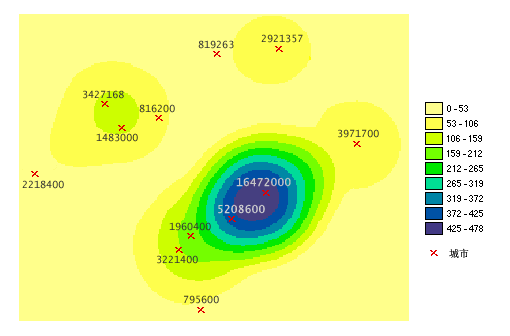“密度分析”工具可以将输入点图层的测量量分布到整个地表上，以生成一个连续的表面。
这里有一个密度分析应用的示例，考虑在某特定地区拥有多家店面的连锁零售店。对于每家店面，管理部门都保存有与顾客有关的销售数字。管理部门假定顾客根据路程的远近来选择光顾哪家店面。在本示例中，很自然地就会假定任何一个顾客总是会选择最近的那家店面。距离最近的店面越远，顾客到那家店面要走的路也就越远。但是离得比较远的顾客也可能光顾其他店面。管理部门想研究顾客居住地点的分布状况。根据这些家店面的销售数字和空间分布情况，管理部门需要将顾客巧妙地分散到整个地表上，以此创建显示顾客分布情况的表面。
点密度分析：用于计算每个输出栅格像元周围的点要素的密度。从概念上讲，每个栅格像元中心的周围都定义了一个邻域，将邻域内点的数量相加，然后除以邻域面积，即得到点要素的密度。
线密度分析：于计算每个输出栅格像元邻域内的线状要素的密度。密度的计量单位为长度单位/面积单位。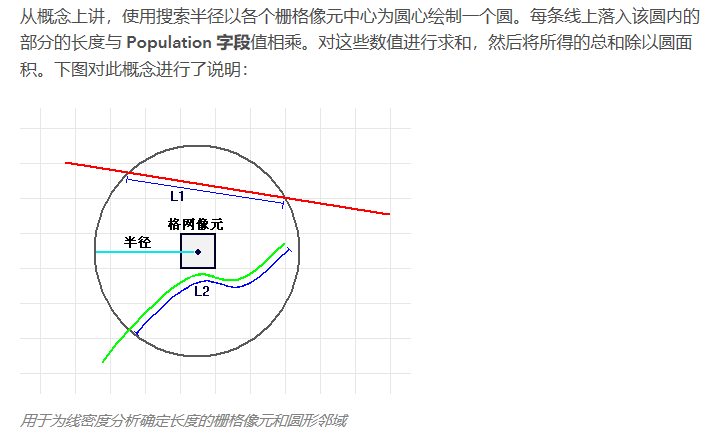核密度分析：使用核函数根据点或折线要素计算每单位面积的量值（根据population字段）以将各个点或折线拟合为光滑锥状表面。
核密度与点密度、线密度分析的区别
点密度工具和线密度工具的区别在于前者适用于点状要素，而后者适用于线状要素。这两种工具均可先计算出已识别邻域内的数量（由 Population 字段指定），然后再将该数量除以邻域的面积。
这两种工具的输出与核密度工具的输出的区别在于，对于点密度和线密度，需要指定一个邻域以便计算出各输出像元周围像元的密度。（可理解为在像元邻域内取点或线均值）
而核密度则可将各点的已知总体数量从点位置开始向四周分散。在核密度中，在各点周围生成表面所依据的二次公式可为表面中心（点位置）赋予最高值，并在搜索半径距离范围内减少到零。对于各输出像元，将计算各分散表面的累计交汇点总数。（可理解为随着与点或线的距离越远，权重越小）

转载于:https://www.cnblogs.com/Ship-chen/p/9887907.html
展开全文• 核密度分析的工作原理：核密度分析工具用于计算要素在其周围邻域中的密度。此工具既可计算点要素的密度，也可计算线要素的密度 可能的用途包括针对社区规划分析房屋密度或犯罪行为，或探索道路或公共设施管线如何...


核密度分析的工作原理：核密度分析工具用于计算要素在其周围邻域中的密度。此工具既可计算点要素的密度，也可计算线要素的密度

可能的用途包括针对社区规划分析房屋密度或犯罪行为，或探索道路或公共设施管线如何影响野生动物栖息地。可使用 population
字段赋予某些要素比其他要素更大的权重，该字段还允许使用一个点表示多个观察对象。例如，一个地址可以表示一栋六单元的公寓，或者在确定总体犯罪率时可赋予某些罪行比其他罪行更大的权重。对于线要素，分车道高速公路可能比狭窄的土路具有更大的影响。

核密度分析用于计算每个输出栅格像元周围的点要素的密度。

概念上，每个点上方均覆盖着一个平滑曲面。在点所在位置处表面值最高，随着与点的距离的增大表面值逐渐减小，在与点的距离等于搜索半径的位置处表面值为零。仅允许使用圆形邻域。曲面与下方的平面所围成的空间的体积等于此点的   Population 字段值，如果将此字段值指定为 NONE 则体积为每个输出栅格像元的密度均为叠加在栅格像元中心的所有核表面的值之和。核函数以 Silverman 的著作（1986 年版，第 76   页，equation 4.5）中描述的四次核函数为基础。

如果 population 字段设置使用的是除 NONE 之外的值，则每项的值用于确定点被计数的次数。例如，值 3
会导致点被算作三个点。值可以为整型也可以为浮点型。

默认情况下，单位是根据输入点要素数据的投影定义的线性单位进行选择的，或是在输出坐标系环境设置中以其他方式指定的。

公式：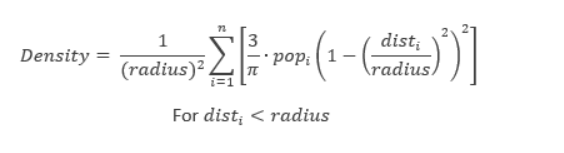详细的信息请到arcgis灌完的在线帮助查看
我这里提供了两种实现的方式，最主要的不同就是点的半径的判断
1.图像长宽小的一边除以30
def KernelDensity(ref_raster, points_shp_file, region_shp, calc_field, out_raster, radius=None, pixel_size=None):
"""
:param ref_raster: 参考栅格
:param points_shp_file: 点shp文件
:param calc_field: 核密度计算字段，表示遍布于用来创建连续表面的景观内的计数或数量。
:param radius: 搜索半径，默认值为输出空间参考中输出范围的宽度或高度的最小值除以 30。
:param pixel_size: 输出的像元大小，默认为参考栅格的像元大小
:return: 核密度结果栅格文件
"""
# 1.点转栅格
ratio_result_file = m_cfg["ratio_result"] + r"/工业GDP系数.tif"
if os.path.exists(ratio_result_file):
return ratio_result_file
raster_from_points = pointsToRaster(points_shp_file, ref_raster, calc_field, pixel_size)
dataset = gdal.Open(raster_from_points, gdalconst.GA_ReadOnly)
dataset_geo_trans = dataset.GetGeoTransform()
x_min = dataset_geo_trans
y_max = dataset_geo_trans
x_res = dataset_geo_trans
col = dataset.RasterXSize
row = dataset.RasterYSize

min_col_row = col if col < row else row
radius = (min_col_row / 30) * x_res if radius == None else radius
# 2.开始计算
raster_data = dataset.GetRasterBand(1).ReadAsArray()
points_xy = []
values = []
point_shp_ds = ogr.GetDriverByName("ESRI Shapefile").Open(points_shp_file, 0)
if point_shp_ds is None:
print("文件{0}打开失败！".format(points_shp_file))
return
points_layer = point_shp_ds.GetLayer(0)
feature_count = points_layer.GetFeatureCount()
for i in range(feature_count):
feature = points_layer.GetFeature(i)
feature_defn = points_layer.GetLayerDefn()
calc_field_index = feature_defn.GetFieldIndex(calc_field)
field_value = feature.GetField(calc_field_index)
values.append(field_value)
point_xy = feature.GetGeometryRef()
points_xy.append([point_xy.GetX(), point_xy.GetY()])

radius_pixel_width = int(radius / abs(x_res))
out_ds = dataset.GetDriver().Create(out_raster, col, row, 1,
dataset.GetRasterBand(1).DataType)  # 创建一个构建重采样影像的句柄
out_ds.SetProjection(dataset.GetProjection())  # 设置投影信息
out_ds.SetGeoTransform(dataset_geo_trans)  # 设置地理变换信息
result_data = np.full((row, col), -999.000, np.float32)  # 设置一个与重采样影像行列号相等的矩阵去接受读取所得的像元值
ratio_data = np.full((row, col), -999.0, np.float32)
region_shp_ds = ogr.GetDriverByName("ESRI Shapefile").Open(region_shp, 0)
region_layer = region_shp_ds.GetLayer(0)
region_feature_count = region_layer.GetFeatureCount()

all_k_points=[]
for i in range(len(points_xy)):

x_pos = int((points_xy[i] - x_min) / abs(x_res))
y_pos = int((y_max - points_xy[i]) / abs(x_res))
# 先搜索以点为中心正方形区域，再在里面搜索圆形
y_len_min = (y_pos - radius_pixel_width - 1) if (y_pos - radius_pixel_width - 1) > 0 else 0
y_len_max = (y_pos + radius_pixel_width + 1) if (y_pos + radius_pixel_width + 1) < row else row

x_len_min = (x_pos - radius_pixel_width - 1) if (x_pos - radius_pixel_width - 1) > 0 else 0
x_len_max = (x_pos + radius_pixel_width + 1) if (x_pos + radius_pixel_width + 1) < col else col
for y in range(y_len_min, y_len_max):
for x in range(x_len_min, x_len_max):
distance = ((x - x_pos) ** 2 + (y - y_pos) ** 2) ** 0.5
# 判断在半径内
if (distance < radius):
value = raster_data[y_pos][x_pos]
scale = (distance * x_res) / radius
D_value = (3 * (1 - scale ** 2) ** 2) / (math.pi * (radius ** 2)) * value
if (result_data[y][x] != -999.0):
result_data[y][x] += D_value
else:
result_data[y][x] = D_value

out_band = out_ds.GetRasterBand(1)
out_band.WriteArray(result_data)
out_band.SetNoDataValue(-999)
out_band.FlushCache()
out_band.ComputeStatistics(False)  # 计算统计信息
out_ds.BuildOverviews('average', [1, 2, 4, 8, 16, 32])  # 构建金字塔

result_data[result_data<0]=0
result_data=result_data/sum(values)
result_data[result_data==0]=-999
array2raster(out_ds, result_data, ratio_result_file, col, row)
return ratio_result_file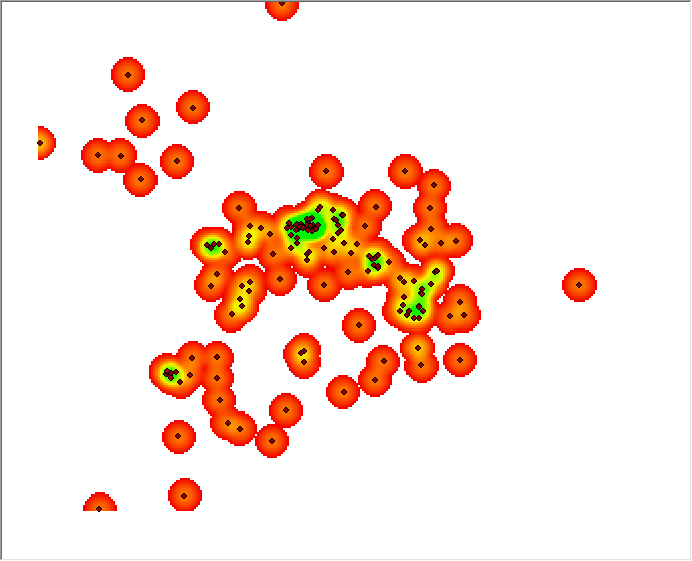2.使用“Silverman 经验规则”的空间变量专为输入数据集计算默认搜索半径（带宽），该变量可有效避免空间异常值（即距离其余点太远的点）。详细的讲解可以看arcgis的在线帮助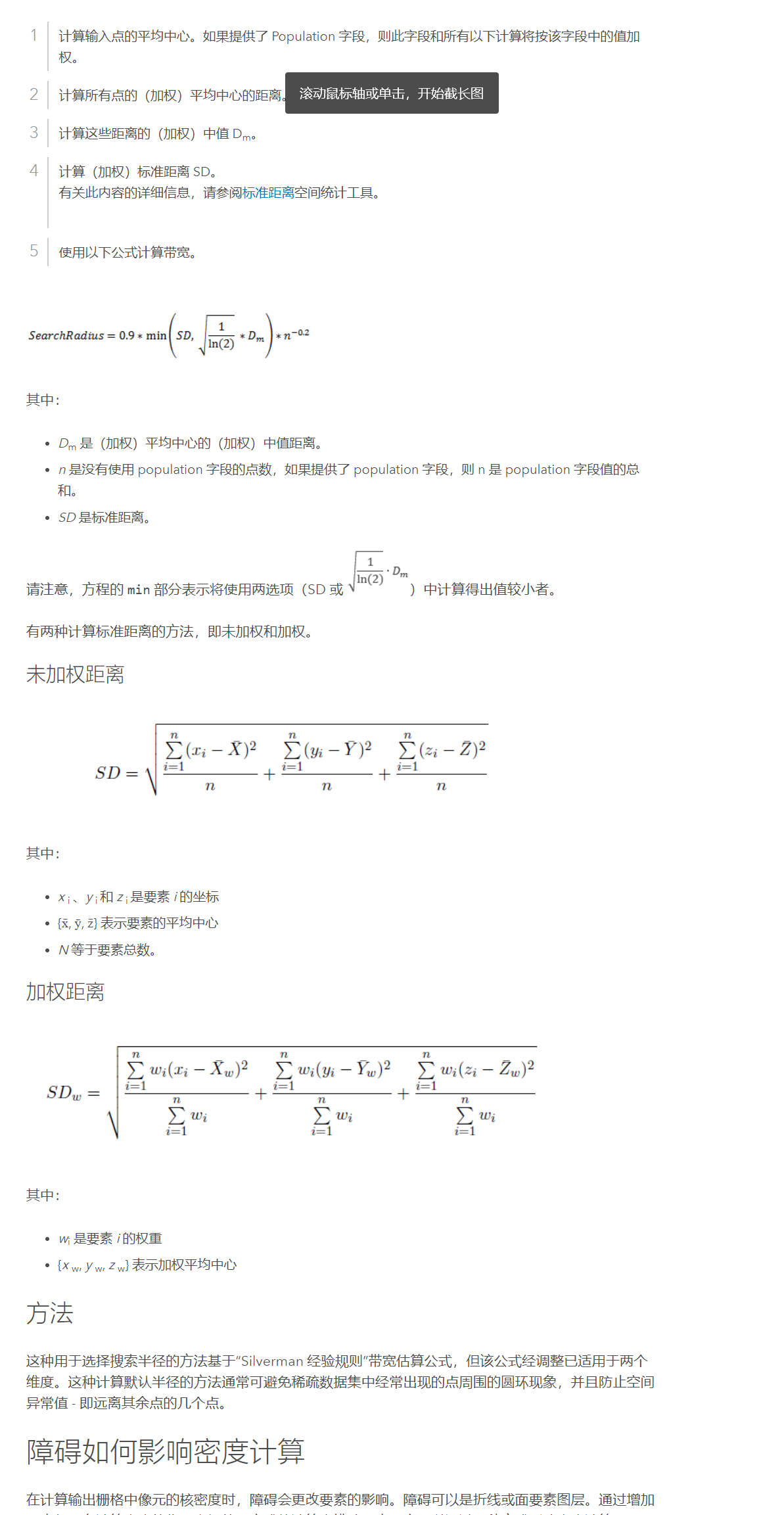def KernelDensity(ref_raster, points_shp_file, calc_field, out_raster, radius=None, pixel_size=None):
"""
:param ref_raster: 参考栅格
:param points_shp_file: 点shp文件
:param calc_field: 核密度计算字段，表示遍布于用来创建连续表面的景观内的计数或数量。
:param radius: 搜索半径，默认值为输出空间参考中输出范围的宽度或高度的最小值除以 30。
:param pixel_size: 输出的像元大小，默认为参考栅格的像元大小
:return: 核密度结果栅格文件
"""
# try:
# 1.点转栅格
raster_from_points = pointsToRaster(points_shp_file, ref_raster, calc_field, pixel_size)
dataset = gdal.Open(raster_from_points, gdalconst.GA_ReadOnly)
dataset_geo_trans = dataset.GetGeoTransform()
x_min = dataset_geo_trans
y_max = dataset_geo_trans
x_res = dataset_geo_trans
print(dataset_geo_trans)
col = dataset.RasterXSize
row = dataset.RasterYSize

min_col_row = col if col < row else row
# 2.开始计算
raster_data = dataset.GetRasterBand(1).ReadAsArray()
points_xy = []
point_shp_ds = ogr.GetDriverByName("ESRI Shapefile").Open(points_shp_file, 0)
if point_shp_ds is None:
print("文件{0}打开失败！".format(points_shp_file))
return
points_layer = point_shp_ds.GetLayer(0)
feature_count = points_layer.GetFeatureCount()
pointXs = []
pointYs = []
values=[]
for i in range(feature_count):
feature = points_layer.GetFeature(i)
feature_defn = points_layer.GetLayerDefn()
calc_field_index = feature_defn.GetFieldIndex(calc_field)
field_value = feature.GetField(calc_field_index)
values.append(field_value)
point_xy = feature.GetGeometryRef()
points_xy.append([point_xy.GetX(), point_xy.GetY()])
pointXs.append(point_xy.GetX()*field_value)
pointYs.append(point_xy.GetY()*field_value)
# 1.计算输入点的平均中心。如果提供了 Population 字段，则此字段和所有以下计算将按该字段中的值加权。
mean_x = sum(pointXs) / len(pointXs)
mean_y = sum(pointYs) / len(pointYs)
# 2.计算所有点的（加权）平均中心的距离。
weight_distances = []
standerd_x = 0
standerd_y=0
for i in range(len(points_xy)):
standerd_x+=(abs(points_xy[i]*values[i] - mean_x)) ** 2
standerd_y+=abs(points_xy[i]*values[i] - mean_y)**2
w_distance = (abs((points_xy[i]*values[i]) ** 2 - mean_x ** 2) + abs((points_xy[i]*values[i]) ** 2 - mean_y ** 2)) ** 0.5
weight_distances.append(w_distance)
# 3.计算这些距离的（加权）中值 Dm。
p_median = np.median(weight_distances)
SDm=math.sqrt(1/np.log(2))*p_median
# 4.计算（加权）标准距离 SD。
SD=(standerd_x/len(points_xy)+standerd_y/len(points_xy)/len(points_xy))**0.5

search_radius=0.9*min(SD,SDm)*((1/len(points_xy))**0.2)
radius = search_radius if radius == None else radius
out_ds = dataset.GetDriver().Create(out_raster, col, row, 1,
dataset.GetRasterBand(1).DataType)  # 创建一个构建重采样影像的句柄
out_ds.SetProjection(dataset.GetProjection())  # 设置投影信息
out_ds.SetGeoTransform(dataset_geo_trans)  # 设置地理变换信息
result_data = np.full((row, col), -999.000, np.float32)  # 设置一个与重采样影像行列号相等的矩阵去接受读取所得的像元值

for i in range(len(points_xy)):
#动态的搜索半径，这里是加权后的搜索半径，
radius_pixel_width=int(search_radius/values[i])
x_pos = int((points_xy[i] - x_min) / abs(x_res))
y_pos = int((y_max - points_xy[i]) / abs(x_res))
# 先搜索以点为中心正方形区域，再在里面搜索圆形
y_len_min = (y_pos - radius_pixel_width - 1) if (y_pos - radius_pixel_width - 1) > 0 else 0
y_len_max = (y_pos + radius_pixel_width + 1) if (y_pos + radius_pixel_width + 1) < row else row

x_len_min = (x_pos - radius_pixel_width - 1) if (x_pos - radius_pixel_width - 1) > 0 else 0
x_len_max = (x_pos + radius_pixel_width + 1) if (x_pos + radius_pixel_width + 1) < col else col

for y in range(y_len_min, y_len_max):
for x in range(x_len_min, x_len_max):
distance = ((x - x_pos) ** 2 + (y - y_pos) ** 2) ** 0.5*values[i]
# 判断在半径内
if (distance < radius):
value = raster_data[y_pos][x_pos]
scale = (distance * x_res) / radius
D_value = (3 * (1 - scale ** 2) ** 2) / (math.pi * (radius ** 2)) * value
if (result_data[y][x] != -999.0):
result_data[y][x] += D_value
else:
result_data[y][x] = D_value

out_band = out_ds.GetRasterBand(1)
out_band.WriteArray(result_data)
out_band.SetNoDataValue(-999)
out_band.FlushCache()
out_band.ComputeStatistics(False)  # 计算统计信息
out_ds.BuildOverviews('average', [1, 2, 4, 8, 16, 32])  # 构建金字塔
del dataset  # 删除句柄
del out_ds
return out_raster

我这里值太大了，搜索半径就会特别的广，这也是正常的，如果你一个点代表了很多的人，肯定分布的区域也会增大很多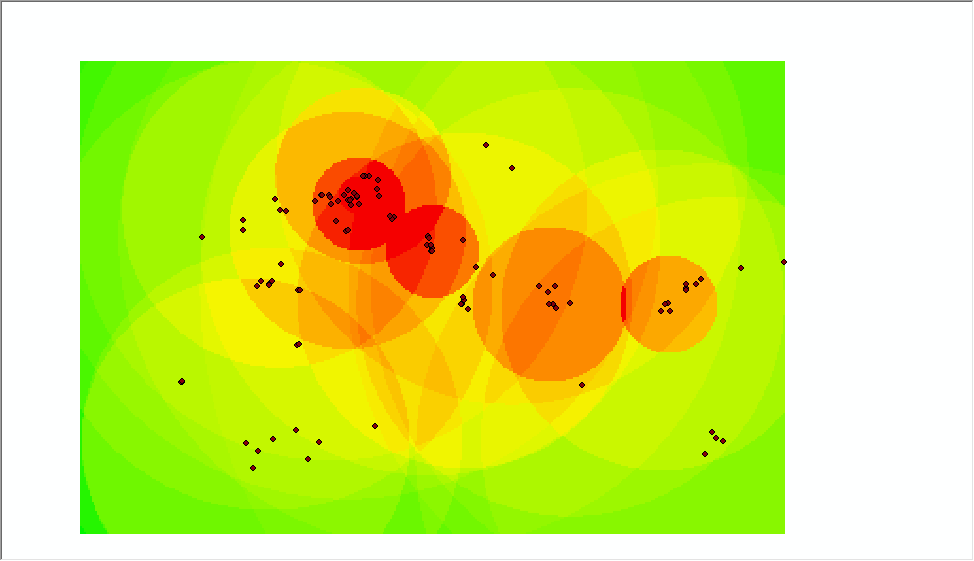# 点转栅格
def pointsToRaster(points_shp_file, in_raster, filed_value, pixel_size=None):
"""
:param points_shp_file: 点shp文件
:param in_raster: 输入参照栅格，一定得是坐标系统为4326的栅格文件
:param filed_value: 赋值字段
:param pixel_size: 输出的像元大小
:return: 结果栅格文件
"""
try:
result_tif = 'result_' + str(uuid.uuid4()) + '.tif'
if pixel_size != None:
in_raster = resampleRaster(in_raster, pixel_size)
dataset = gdal.Open(in_raster, gdalconst.GA_ReadOnly)

geo_transform = dataset.GetGeoTransform()
cols = dataset.RasterXSize  # 列数
rows = dataset.RasterYSize  # 行数

shp = ogr.Open(points_shp_file, 0)
m_layer = shp.GetLayerByIndex(0)
out_file = m_cfg["temp_path"] + r"/toraster_{0}.tif".format(str(uuid.uuid4()))
target_ds = gdal.GetDriverByName('GTiff').Create(out_file, xsize=cols, ysize=rows, bands=1,
eType=gdal.GDT_Float32)
target_ds.SetGeoTransform(geo_transform)
target_ds.SetProjection(dataset.GetProjection())

band = target_ds.GetRasterBand(1)
band.SetNoDataValue(-999)
band.FlushCache()
gdal.RasterizeLayer(target_ds, , m_layer, options=["ATTRIBUTE=" + filed_value])  # 跟shp字段给栅格像元赋值
del dataset
shp.Release()
print('转栅格完成！')
return out_file
except Exception as e:
print("转栅格出错:{0}".format(str(e)))
return


# 重新设置栅格像元大小
def resampleRaster(in_raster, pixel_size):
"""
:param in_raster: 输入栅格
:param pixel_size: 输出的像元大小
:return: 结果栅格文件
"""
try:
in_ds = gdal.Open(in_raster)
out_raster = m_cfg["temp_path"] + r"/resample_{0}.tif".format(str(uuid.uuid4()))
geotrans = list(in_ds.GetGeoTransform())
ratio = geotrans / pixel_size
geotrans = pixel_size  # 像元宽度变为原来的两倍
geotrans = -pixel_size  # 像元高度也变为原来的两倍
in_band = in_ds.GetRasterBand(1)
xsize = in_band.XSize
ysize = in_band.YSize
x_resolution = int(xsize * ratio)+1  # 影像的行列都变为原来的一半
y_resolution = int(ysize * ratio)+1
out_ds = in_ds.GetDriver().Create(out_raster, x_resolution, y_resolution, 1,
in_band.DataType)  # 创建一个构建重采样影像的句柄
out_ds.SetProjection(in_ds.GetProjection())  # 设置投影信息
out_ds.SetGeoTransform(geotrans)  # 设置地理变换信息
data = np.empty((y_resolution, x_resolution), np.float32)  # 设置一个与重采样影像行列号相等的矩阵去接受读取所得的像元值
in_band.ReadAsArray(buf_obj=data)
out_band = out_ds.GetRasterBand(1)
out_band.WriteArray(data)
out_band.SetNoDataValue(-999)
out_band.FlushCache()
out_band.ComputeStatistics(False)  # 计算统计信息
out_ds.BuildOverviews('average', [1, 2, 4, 8, 16, 32])  # 构建金字塔
del in_ds  # 删除句柄
del out_ds
print("重设栅格大小成功")
return out_raster
except Exception as e:
print("重设栅格大小出错{0}".format(str(e)))
return


# 根据计算后的数组生成tif
def array2raster(dataset, data, out_file, x_size, y_size):
if os.path.exists(out_file):
return out_file
out_ds = dataset.GetDriver().Create(out_file, x_size, y_size, 1,
dataset.GetRasterBand(1).DataType)  # 创建一个构建重采样影像的句柄
out_ds.SetProjection(dataset.GetProjection())  # 设置投影信息
out_ds.SetGeoTransform(dataset.GetGeoTransform())  # 设置地理变换信息
out_band = out_ds.GetRasterBand(1)
out_band.WriteArray(data)
out_band.SetNoDataValue(-999)
out_band.FlushCache()
out_band.ComputeStatistics(False)  # 计算统计信息
out_ds.BuildOverviews('average', [1, 2, 4, 8, 16, 32])  # 构建金字塔
del dataset  # 删除句柄
del out_ds
return out_file




展开全文python
• 核密度分析工具用于计算要素在其周围邻域中的密度。此工具既可计算点要素的密度，也可计算线要素的密度。核密度分析可用于测量建筑密度、获取犯罪情况报告，以及发现对城镇或野生动物栖息地造成影响的道路或公共设施...
• 针对混响背景中的动目标检测问题，将基阵接收数据经过波束形成与匹配滤波后的输出视作统计观测空间，基于背景和目标回波在该空间中的统计特性差异，采用非参量核密度函数估计方法构造ping 情况下的检验统计量，...人工智能
• sm包中sm.density.compare函数用于绘制核密度图，核密度图是用一条密度曲线而不是通过柱状来展示连续型变量的分布。相比直方图，密度图的一个优势是可以堆放，可用于比较组间差异。sm.density.compare函数可以直接...
关注公众号凡花花的小窝，收获更多的考研计算机专业编程相关的资料
绘制其他图形
核密度图
sm包中sm.density.compare函数用于绘制核密度图，核密度图是用一条密度曲线而不是通过柱状来展示连续型变量的分布。相比直方图，密度图的一个优势是可以堆放，可用于比较组间差异。sm.density.compare函数可以直接堆放多条密度曲线。使用格式如下。
sm.density.compare(x，group，…)
其中x是数值向量，group是分组向量，是因子型数据。
sm.density.compare函数
corrgram(x,order=, lower.panel= ,      upper.panel=,text.panel=,diag.panel=,…)
示例：mtcars数据集wt的核密度图

library(sm)      # 加载sm包
sm.density.compare(mtcars$wt, factor(mtcars$cyl))     # 绘制核密度图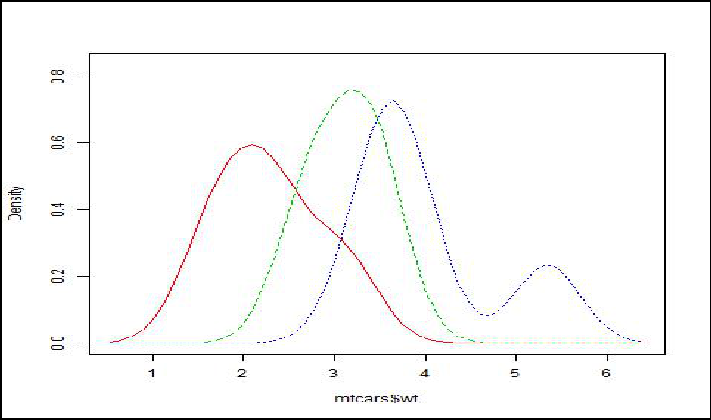（mtcars$wt,factor(mtcars$cyl))

小提琴图
vioplot包中的vioplot函数用于绘制小提琴图，小提琴图是核密度图与箱线图的结合，本质是利用密度值生成的多边形，但该多边形同时还沿着一条直线作了另一半对称的“镜像”，这样两个左右或上下对称的多边形拼起来就形成了小提琴图的主体部分，最后一个箱线图也会被添加在小提琴的中轴线上。使用格式如下。
vioplot( x, …, range=1.5, h, ylim, names, horizontal=FALSE, …)
示例：mtcars数据集wt的小提琴图

library(vioplot)      # 加载vioplot包
attach(mtcars)
par(mfrow = c(2, 1))
vioplot(wt[cyl4], wt[cyl6], wt[cyl==8],  border=“black”, col = “light green”, rectCol = “blue”, horizontal = TRUE)  # 绘制小提琴图
title(main = ‘小提琴图’)  # 添加标题
boxplot(wt~cyl, main = ‘箱线图’, horizontal=TRUE, pars=list(boxwex=0.1), border=“blue”)  # 绘制箱线图
par(mfrow = c(1, 1))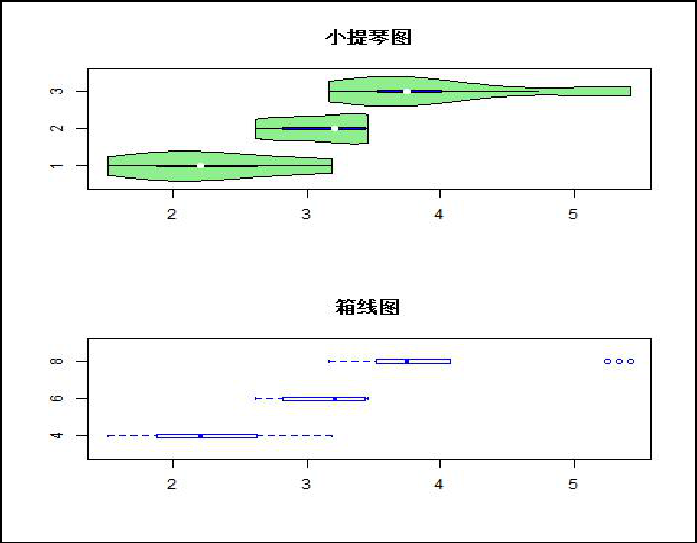QQ图
qqplot函数用于绘制QQ图，QQ图检查数据是否服从某种分布。QQ图的原理是如果一批数据服从某种理论分布，看其经验分布和理论分布是否一致。将排序后的数据和理论分布的分位数进行比较后大致相等，说明了经验分布和理论分布相似。使用格式如下。
qqplot(x, y,…); qqnorm(y,…);qqline(y)
qqplot(x,y,…);qqnorm(y,…);qqline(y)
示例：mtcars数据集wt的QQ图

qqnorm(wt)     #正态分布QQ图
qqline(wt)      #QQ线
qqplot(qt(ppoints(length(wt)), df = 5), wt,xlab = “Theoretical Quantiles”, ylab = “Sample Quantiles”, main = “Q-Q plot for t dsn”)       #t分布QQ图
qqline(wt)      #QQ线

qqline(wt),
qqplot(qt(ppoints(length(wt)),df=5),wt,lab=“Theoretical Quantiles”,ylab=“Sample Quantiles”,main=“Q-Q plot for t dsn”)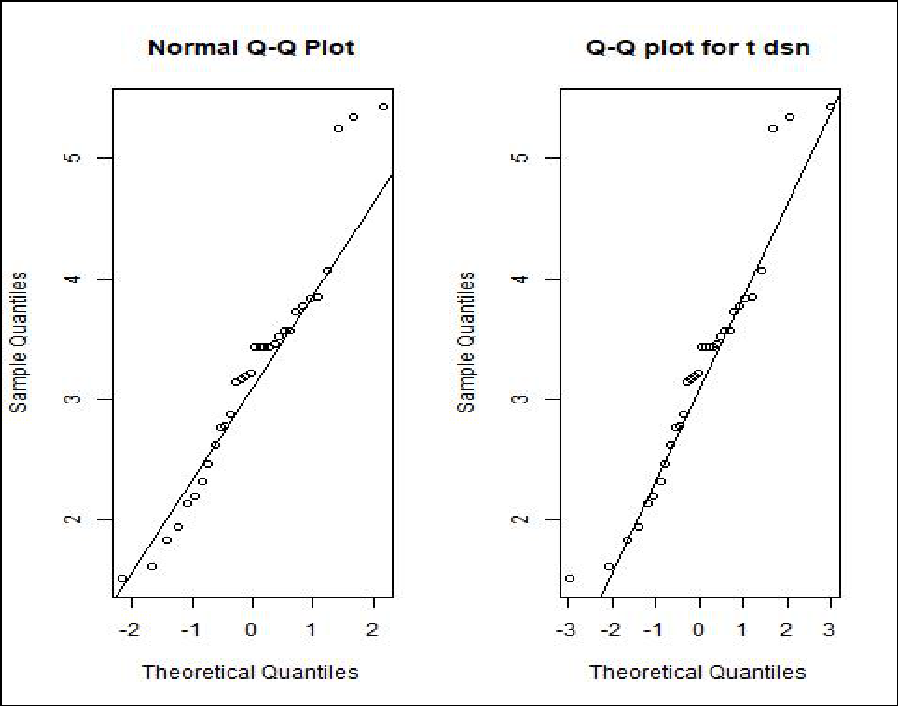星状图
stars函数用于绘制星状图，星状图用线段离中心的长度来表示变量值的大小，展示多变量个体，每个变量的图形相互独立，整幅图形看起来就像很多星星。使用格式如下。
stars(x,full=TRUE,scale=,radius=,labels=,locations=,flip.labels=,draw.segments=,col.segments=,col.stars=, frame.plot=,…)
参数解释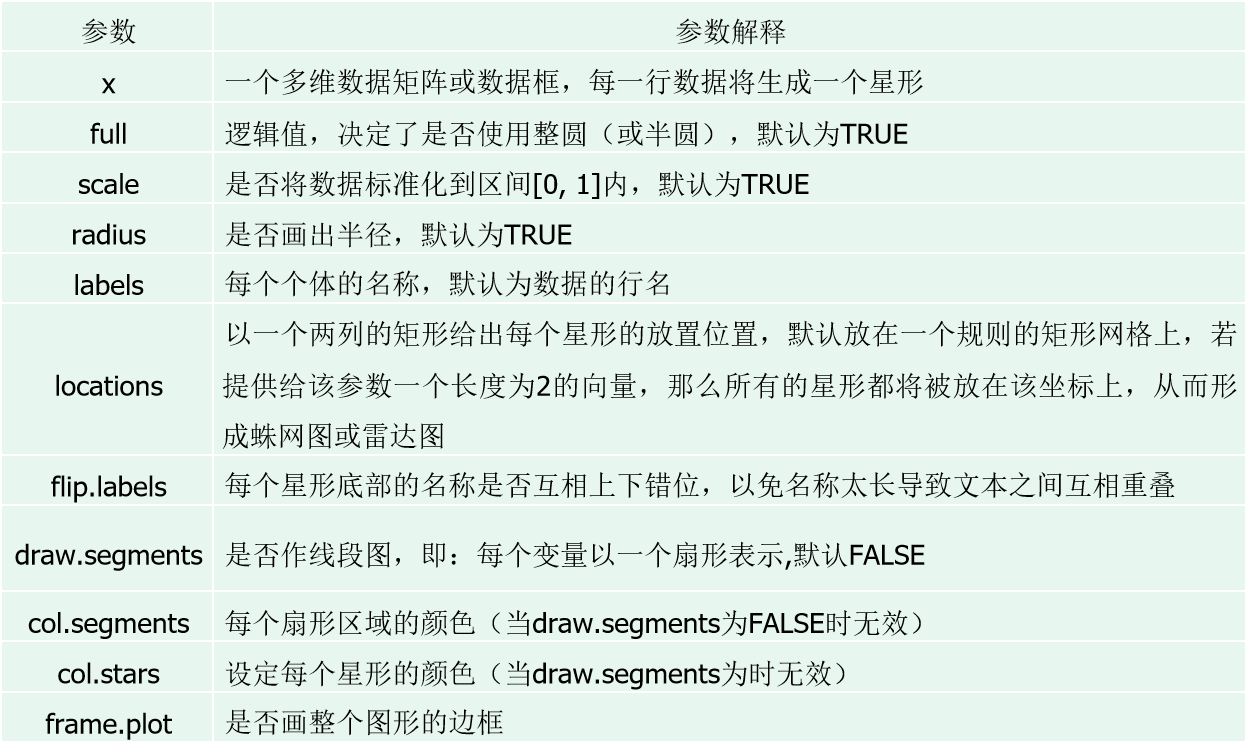x
一个多维数据矩阵或者数据框，每一行数据将会生成一个星型
fulll
逻辑值，据定了是否使用整圆或者半圆，默认为TRUE
scale
是否讲数据标准化到区间[0,1]内，默认为TRUE
radius
是否画出半径，默认为TRUE
labels
每一个个体的名称，默认为数据的行名
draw.segments
是否做出线段图，即：每一个变量以一个扇形标识，默认为FALSE
col.segments每一个扇形区域的颜色
draw.segments为FALSE的时候无效
col.stars设定每一个星型的颜色
frame.plot是否画出整个图形的边框

stars(mtcars, draw.segments = TRUE)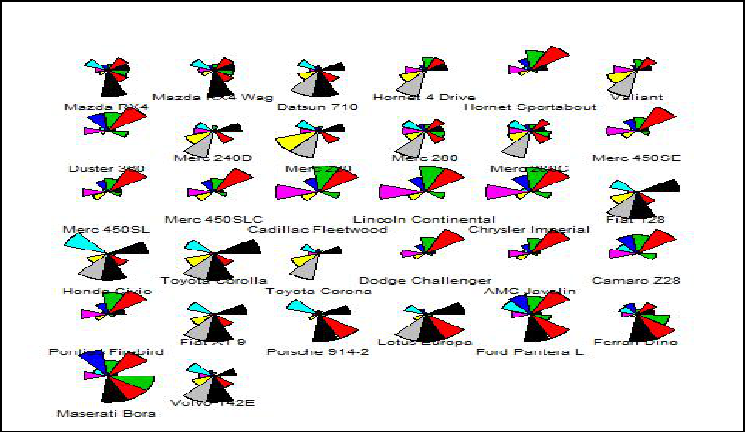等高图
contour函数用于绘制等高图，等高图所展示数据的形式是两个数值向量x、y和一个相应的矩阵z。x、y交叉组合之后形成的是一个“网格”，z是这个网格上的高度数值，将平面上对应的z值（高度）相等的点连接起来形成的线就是等高线。对x、y进行核密度估计，得到一个密度值矩阵，然后用x、y以及这个密度值矩阵作等高图。由于密度值反映的是某个位置上数据的密集程度，等高图就展示了一个聚类现象。 使用格式如下
contour(x=,y=,z,nlevels=,levels=,labels= ,method=,...)

contour函数参数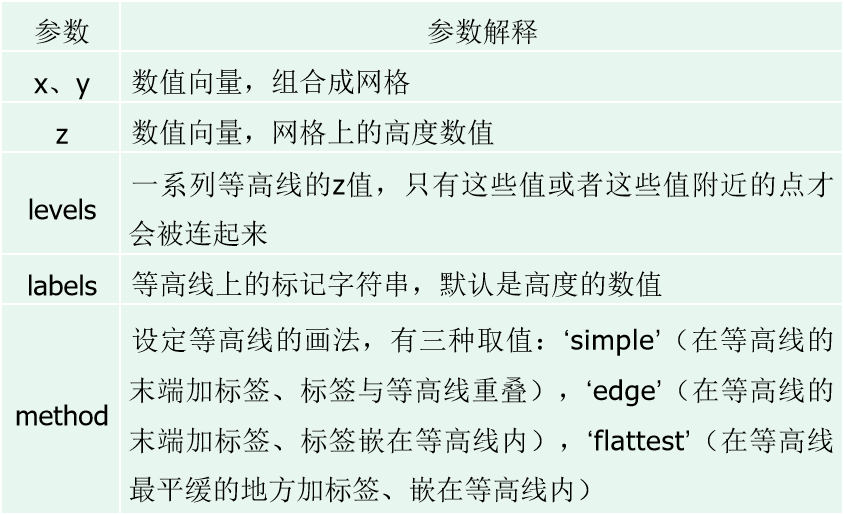flattest:在等高线最平缓的地方加标签，嵌在等高线内
edge在等高线的末端加标签，标签嵌在等高线内
simple在等高线的末端加标签，标签与等高线重叠
示例：mtcars数据集wt与mpg的等高线图

library(KernSmooth)  # 计算二维核密度的包
mtcars1 = data.frame(wt, mpg)
est = bkde2D(mtcars1, apply(mtcars1, 2, dpik))     # 计算二维核密度
contour(est$x1, est$x2, est$fhat, nlevels = 15, col = “darkgreen”, xlab = “wt”, ylab = “mpg”) # 画等高图 points(mtcars1) # 添加散点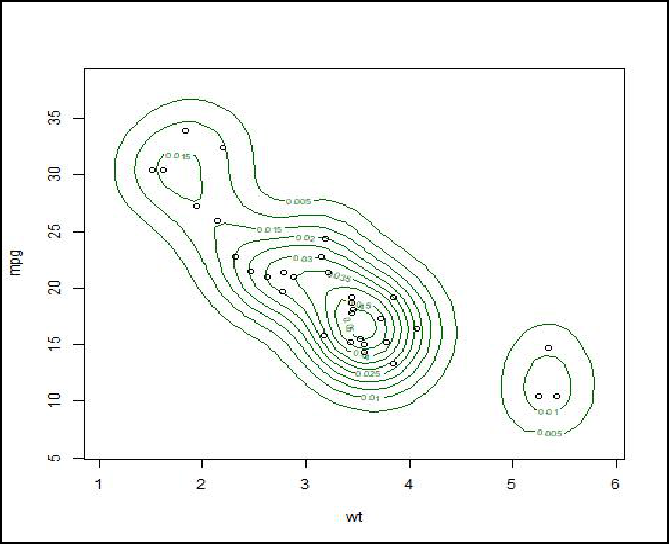2.修改图形参数 R是一个功能强大的图形构建平台,可以通过逐条输入语句构建图形元素（颜色、点、线、文本以及图例等），逐渐完善图形特征，直至得到想要的效果。图形元素的显示可以用图形函数和绘图参数来改良，也可以用绘制图形元素的基础函数来控制。 修改颜色 固定颜色选择函数 R语言提供了自带的固定种类的颜色，主要涉及的是**colors函数，该函数可以生成657种颜色名称，代表657种颜色，**可以通过以下代码 查看R自带颜色的前20种颜色的名称。 colors()[1:20] #查看前20种颜色  “white” “aliceblue” “antiquewhite” “antiquewhite1”  “antiquewhite2” “antiquewhite3” “antiquewhite4” “aquamarine”  “aquamarine1” “aquamarine2” “aquamarine3” “aquamarine4”  “azure” “azure1” “azure2” “azure3”  “azure4” “beige” “bisque” “bisque1” 打印657种颜色 op=par(mfrow = c(length(colors())%/%60 + 1, 1)) # 画布分割 par(mar=c(0.1,0.1,0.1,0.1), xaxs= “i”, yaxs = “i”) #设置画布的边距和坐标轴的样式 for(i in 1:(length(colors())%/%60 + 1)){ barplot(rep(1,60), col=colors()[((i-1)60+1):(i60)], border = colors()[((i - 1)60 + 1):(i60)]) box() # 加边框 } par(op) 整除,取小数点前面的数字。比如5%/%2 会得到2 mar： 行边距（下 左 上 右） xaxt/yaxt：坐标轴的样式，默认值为s，表示以标准样式显示。 axes：logical. If TRUE, a vertical (or horizontal, if horiz is true) axis is drawn. mar行边距，xaxt/yaxt坐标轴的样式，默认值为s表示以标准样式显示 打印657种颜色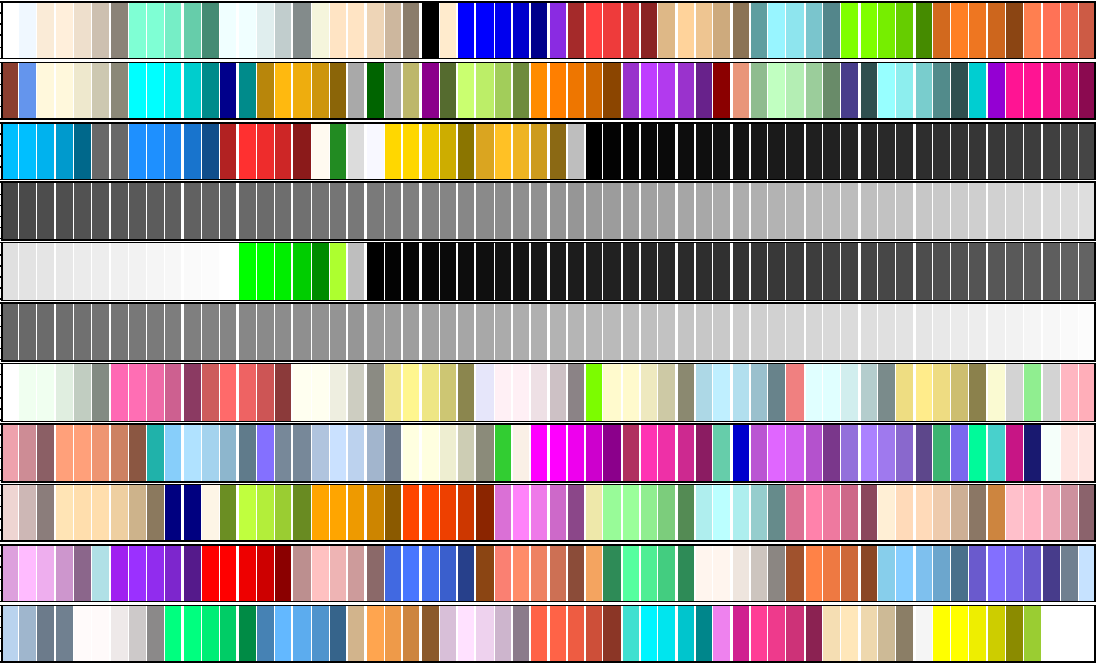固定颜色选择函数 通过palette函数固定调色板，只要设定好了调色板，它的取值就不会再改变（直到下一次重新设定调色板）。 palette() #返回当前的调色板设置，此时为默认值  “black” “red” “green3” “blue” “cyan” “magenta” “yellow” “gray” palette(colors()[1:10]) #重新设置调色板为colors的前10种颜色 palette() #返回当前的调色板设置，此时为colors()的前10种颜色  “white” “aliceblue” “antiquewhite” “antiquewhite1” “antiquewhite2” “antiquewhite3”  “antiquewhite4” “aquamarine” “aquamarine” “aquamarine2” palette(‘default’) #恢复默认的调色板设置 固定颜色选择函数 调色板的好处在于设置绘图函数的col参数时，可以直接用一个整数来表示颜色，这个整数对应的颜色就是调色板中相应位置的颜色。若整数值超过了调色板颜色向量的长度，那么R会自动取该整数除以调色板颜色向量长度的余数。即在绘图函数中，col=i等价于col=palette()[i]。 col=i等价于col=palette()[i]. 以iris数据集为例，使用plot函数绘制Sepal.Length与Sepal.Width的散点图时，可以在不同Species使用不同的颜色绘制散点，以便区分种类。 plot(iris$Sepal.Length, iris$Sepal.Width, col = c(1:3)) 等价于 plot(iris$Sepal.Length, iris$Sepal.Width, col = iris$Species)

Species为因子型数据,setosa versicolor virginica分别对应数字1，2，3，即等价于col = rep(1:3, each = 50)plot(iris$Sepal.Length, iris$Sepal.Width, col = 2)
渐变色生成函数
除了固定颜色选择函数外，R还提供了一系列渐变颜色生成函数，这些函数用来控制颜色值逐步变化。其中，主要的渐变色生成函数如表 。
渐变颜色生成函数。这些函数用来控制颜色值逐步变化，其中，主要的渐变色生成函数如表，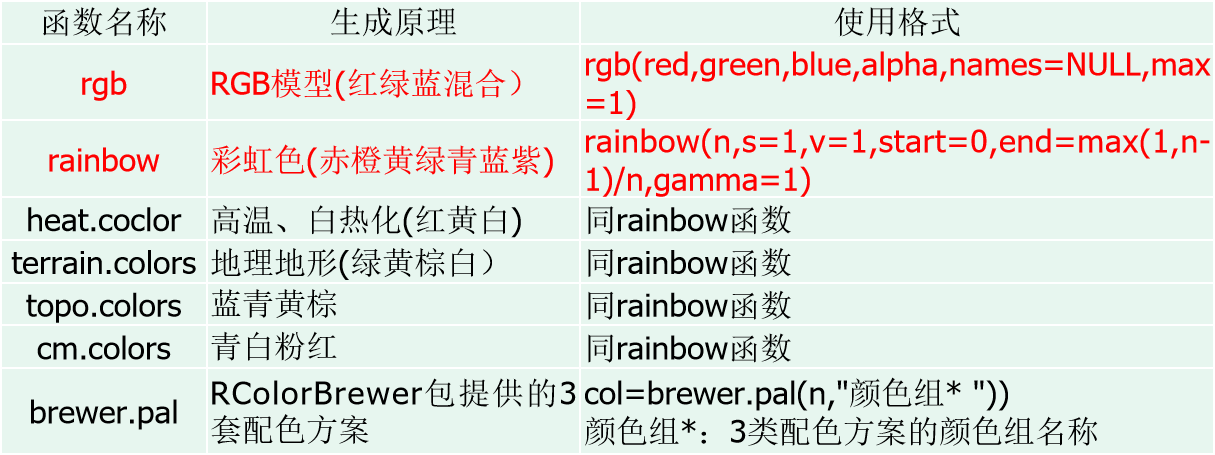heat.coclor高温，白热化（红黄白）
terrain.colors地理地形
topo.colors蓝青黄粽
cm.colors蓝青黄粽
cm.colors青白粉红
rgb函数把RGB颜色转化为十六进制数值，使用格式前四个参数都取值于区间[0, max]，names参数用来指定生成颜色向量的名称。red，green，blue参数的值越大就说明该颜色的成分越高。alpha指的是颜色的透明度，取0表示完全透明，取最大值表示完全不透明（默认完全不透明）。
rainbow函数、heat.coclor函数、terrain.colors函数、topo.colors函数、cm.colors函数是主题配色函数，使用格式中n设定产生颜色的数目，start和end设定彩虹颜色的一个子集，生成的颜色将从这个子集中选取。
关于颜色的调配：
https://www.cnblogs.com/xianghang123/archive/2012/06/13/2547992.html
red,green,blue参数的值越大就说明干颜色的成分越高，aipha指的是颜色的透明度
rainbow函数，heat.coclor函数，terrain.colors函数
主题调色板-rainbow( )
R本身也提供特定颜色主题的配色方案，这些配色方案用一系列渐变的颜色表现 的主题。
rainbow（）即彩虹的颜色，是由“赤、橙、黄、绿、青、蓝、紫”一系列颜色组成的。该函数用法如下：
Rainbow（n,s=1,v=1,start=0,end=max(1,n-1)/n,gamma=1）;
n：设定产生颜色的数目。
start和end：设定彩虹颜色的一个子集，生成的颜色将从这个子集中选取。
rainbow(n,s=1,v=1,start=0,end=max(1,n-1)/n,gamma=1);
barplot(rep(1,41),
col=c(rainbow(n=6,start = 0,end = 1/6),“white”,
rainbow(n=6,start=1/6,end = 2/6),“white”,
rainbow(n=6,start=2/6,end = 3/6),“white”,
rainbow(n=6,start =3/6,end=4/6 ),“white”,
rainbow(n=6,start = 4/6,end = 5/6),“white”,
rainbow(n=6,start=5/6,end=1)))
x.text<-c(“赤”,“黄”,“绿”,“青”,“蓝”,“紫”)
axis(side=1,at=seq(from=3,length.out = 6,by=8.5),labels = x.text,cex=0.75)
渐变色生成函数调色板的颜色样式
rgb<-rgb(red=255,green=1:255,blue=0,max=255)
par(mfrow=c(6,1))
par(mar=c(0.1,0.1,2,0.1), xaxs=“i”, yaxs=“i”)   #坐标轴计算方式
barplot(rep(1,255),col= rgb,border=rgb,main=“rgb”)
barplot(rep(1,100),col=rainbow(100),border=rainbow(100),main=“rainbow(100))”)
barplot(rep(1,100),col=heat.colors(100),border=heat.colors(100),main=“heat.colors(100))”)
barplot(rep(1,100),col=terrain.colors(100),border=terrain.colors(100),main=“terrain.colors(100))”)
barplot(rep(1,100),col=topo.colors(100),border=topo.colors(100),main=“topo.colors(100))”)
barplot(rep(1,100),col=cm.colors(100),border=cm.colors(100),main=“cm.colors(100))”)
渐变色生成函数调色板的颜色样式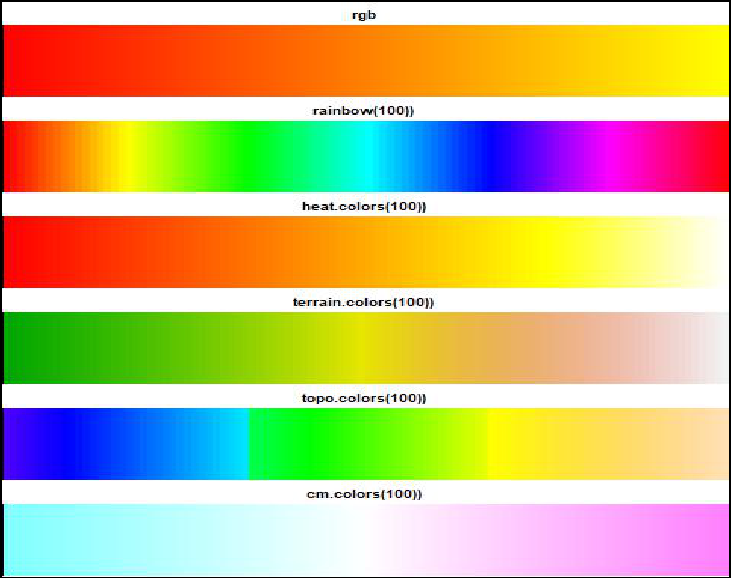RColorBrewer包
RColorBrewer包提供了3套配色方案，分别为连续型，极端型以及离散型。
连续型（Sequential）指生成一系列连续渐变的颜色，通常用来标记连续型数值的大小。共18组颜色，每组分为9个渐变颜色展示。
极端型（Diverging）指生成用深色强调两端、浅色标示中部的系列颜色、可用来标记数据中的离群点。共9组颜色，每组分为11个渐变颜色展示。
离散型（Qualitative）指生成一系列彼此差异比较明显的颜色，通常用来标记分类数据。共8组颜色，每组渐变颜色数不同。
RColorBrewer包三种类型的颜色展示
par(mfrow = c(1,3))
library(RColorBrewer)
par(mar=c(0.1,3,0.1,0.1))
display.brewer.all(type=“seq”)
display.brewer.all(type=“div”)
display.brewer.all(type=“qual”)
RColorBrewer包三种类型的颜色展示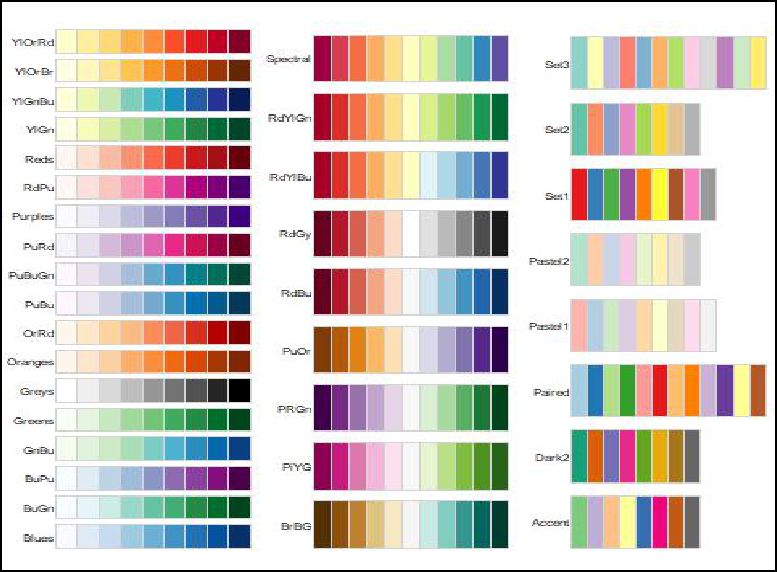RColorBrewer包及rainbow函数绘制颜色的散点图
左图
library(RColorBrewer)
my_col <- brewer.pal(3, ‘RdYlGn’) # brewer.pal(n, name),其中n为颜色的数量，name表示颜色组的名称
plot(iris$Sepal.Length, iris$Sepal.Width, col = rep(my_col, each =50))
右图
plot(iris$Sepal.Length, iris$Sepal.Width, col = rep(rainbow(3), each = 50))
RColorBrewer包及rainbow函数绘制颜色的散点图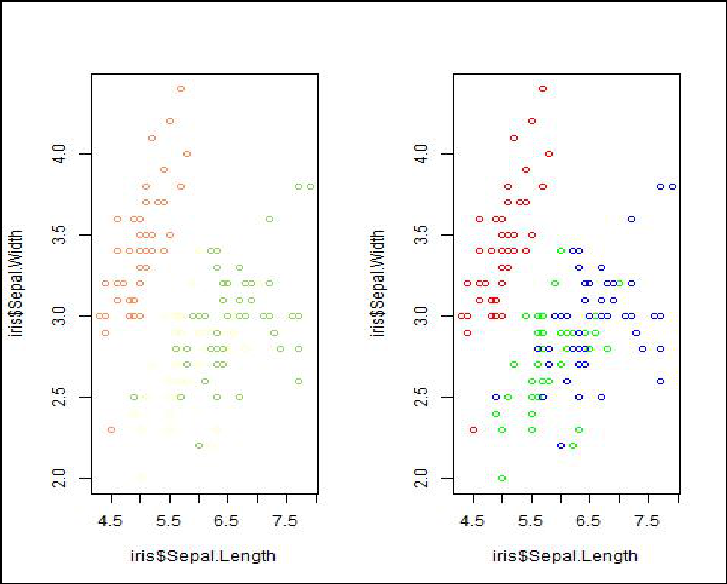展开全文• 提出了一种用于轴向连接纳米线核-壳pn结的高效太阳能电池的复合纳米结构。... 通过出色的电流匹配，在0.283（纳米线阵列的密度）的低填充率下（比串联轴向pn结要高得），可以实现19.9％的有希望的效率。
• 层数越，就可以把线间距布得越大，使路径选择更容易，而且减少了串扰问题的风险。遗憾的是，多层印刷电路板的费用与层的数字和表面面积的乘积成正 比。使用层数越，费用也就越高。 如果层数减少，必须使用更小的...
层数越多，就可以把线间距布得越大，使路径选择更容易，而且减少了串扰问题的风险。遗憾的是，多层印刷电路板的费用与层的数字和表面面积的乘积成正 比。使用层数越多，费用也就越高。 如果层数减少，必须使用更小的走线间距，那同样也将增加额外的费用。不仅如此，对于正好足够的走线间距，所冒的串扰风险太大。 决定一个板子需要的最少层数，靠的是经验和猜测相结合。
问题的核心是：在一个确定大小的线路板上，使用M层，布通N个连接，估算所需要的走线间距，知道了走线间距，就能知道板子费用，而且同时可以给出串扰模型。
走线间距由线路密度决定。关于线路密度，有个很有用的模型，称为RENT准则，是以推广它的IBM工程师的名字命名的。RENT注意到，大多数正方 形板子，当分为四个象限时，一半接线在象限之间，一半接线在每个象限内部。进一步细分每个象限，显示出相同分布。关于在两个象限之间的走线，我们假设接线 的长度平均起来等于象限之间 的间距，则接线的总平均等于板子边沿的3/8。
知道了线的平均长度和线的数目，可以计算出这些线使用任意走线间距时所占的总面积。下式给出了一个使用M层的固定大小的板子上走通N个连接所需的走线间距。 当然，如果我们还不其他一些关于布线需求的信息，例如大的总线或其他的结构，则应该使用它，如果没有其他信息。可以尝试使用RENT的思想，计算在一个板子上走线时所需的空间：
其中：N=连接的数目 P平均=平均走线间距，IN X=板宽度，IN Y=板高度，IN M=走线的层数 举例来说，一个8IN*12IN的板子，在四个层面上共有800个走线连接。我们需要的走间距平均为0.132IN。 这意味着，如果板子几乎被双列直播的通孔所覆盖，几乎需要在每个引脚之间进行走线。 不要指望能占用引脚之间超过一半的空间。在上述例子中，我们应该使用更多的层，或者使用双倍走线路径。
对于通孔板，与由上式得出的平均间距和最小间距的需求完全不同，如果需要在引脚之间使用两倍或三倍的走线路径，应该从串扰的角度考虑决定所使用的最小间距。采用由上式得出平均间距，可以确定需要多少可用的走线路径。 表面贴装的板子比双列直播的板子的内层有更多可利用的走线空间。通孔的总数大致是相同的，但是表面贴装设计的通孔要小一些，因为集成电路的引脚不需要穿过它们。表面贴装的板子的内层，平均间距和最小间距可能是相近的。
在环氧树脂电路板的内层，引脚之间走四条线是可能的，但是这也可能引起严重的串扰问题。 在芯片间留出大的空间可以在板子上得到额外的走线空间，但是这需要更多的总面积。大多数的设计者会选择增加层数。 如果串扰成为必须考虑的问题，则要保证布线时只有当需要穿过引脚之间时才把走线推挤到一起，一旦它们走向下一个芯片，立刻把它们的展开，这需要许多手动高速，但是即使走线间隙的微小增加也会使串把减少。 带一些运气，我们也能找出适合的电路板走线层数，使其产生的串扰是可接受的，而且不需要太多费用。
展开全文• R包hdrcde提供了用于在一维和二维中计算最高密度区域的工具，以一个协变量为条件的单变量密度函数的估计以及峰回归的工具。 该软件包实现了以下论文中描述的方法。 Richard J Samworth和Matthew P ...R
• 密度服务器很可能会增加单个桌面的成本，还不支持更高级的...和传统的服务器相比，高密度服务器能够在更小的物理空间内提供更的计算能力。例如，Dell高度为2U的PowerEdge FX产品线能够支持高达128个计算核心。...
• 2、圈层集聚是中国经济密度演化的主导趋势3、沿海化是中国经济密度增长的重要景观4、“两纵两横”的轴线分布是中国产业密度集聚的主要承载区域5、中国经济密度分布具有显著的中心特征，形成层次的城市等级体系。...
• 分析了以圆滑约束最小二乘法为核心的反演程序,并通过实例验证了三维高密度电法正、反演计算方法的正确性、准确性,三维立体成图的直观性。该方法提高了对矿井老空区分布范围及富水性的探测能力,为控制矿井突水事故...
• XXX的维度有时比监督学习中高很，我们关心的性质经常比简单的位置估计μ(x)\mu (x)μ(x)复杂得．不过因素在某种程度上被缓解，原因在于我们不需要在其它变量的值改变的情况下推断P(X)P(X)P(X)的性质怎么改变．...聚类 无监督学习 降维 Apriori pagerank算法
• 为充分利用遥感影像的波段光谱特征，提高水边线的提取精度，提出了基于主元分析（KPCA）光谱特征约束的水边线提取模型。利用KPCA变换提取水体样本的光谱特征，采用最大似然法估计特征空间中水体光谱特征概率密度...
• 核密度方法分别建立了图像颜色与纹理特征的概率密度模型，并用贝叶斯模型估计后验概率作为像素能量。构造的新曲线区域能量泛函分别统计内部能量与外部能量之和，以最小能量曲线对应跟踪目标的曲线。通过计算梯度...贝叶斯模型 水平集
• 核心板，顾名思义，即硬件构成中关键的器件和电路打包封装的一块电子主板，具有布线复杂、多层、高频信号干扰、器件密度高等特性，大多数核心板集成了处理器、内存、存储器、电源管理和引脚，通过引脚与配套基板连接...嵌入式 机器学习 物联网 操作系统 人工智能
• 我们之前学习过使用jointplot()来表现双变量数据分布，不过今天，我们将学习一下如何使用基于核密度估计的等高线图来表现双变量数据分布。 我们先来看看代码和图形效果： import numpy as np import seaborn as ...
• 混合高斯模型（Gaussian Mixture Model，简称GMM）是用高斯概率密度函数（正态分布曲线）精确地量化事物，将一个事物分解为若干的基于高斯概率密度函数（正态分布曲线）形成的模型。通俗点讲，无论观测数据集如何...可视化 聚类 机器学习 python 数据挖掘
• （一）、有时候数据太太集中，散点图上的信息不容易看出来，例如： > x <- rnorm(10000, 0, 1)>...首先使用MASS程序包中的二维核密度函数kde2d()来估计二维数据的密度函数，再利用函数contour（）画出密...
• Cisco 3800系列路由器具有内嵌安全处理、大幅系统和内存优化以及全新高密度接口，可在要求最严格的企业环境中提供扩展关键任务安全性、IP电话、商业视频、网络分析和Web应用所需的性能、可用性和可靠性。Cisco 3800...网络
• 小提琴图是箱线图与核密度图的结合，箱线图展示了分位数的位置，核密度图则展示了任意位置的密度，通过小提琴图可以知道哪些位置的数据点聚集的较，因其形似小提琴而得名。 其外围的曲线宽度代表数据点分布的密度...
• 对于单变量的数据来说，采用直方图或核密度曲线是个不错的选择，对于双变量来说，可采用面板图形展现，比如散点图、二维直方图、核密度估计图形等。针对这种情况，Seaborn库提供了对单变量和双变量分布的绘制函数...python 数据分析 数据可视化
• VESTA是结构模型的三维可视化程序，体积数据如电子/核密度和晶体形貌。我这里有 晶体结构可视化软件VESTA入门教程，见与你有缘，就分享给你啦，让你也能轻松绘制出如图1所示的漂亮的晶体/材料结构图。 软件简介 墨迹...
• 最佳实践组网 图 1 数据中心二层网流结构核心/接入组网 图 2 数据中心三层...图 7 服务器集中存储解决方案组网 图 8 双机双阵列存储集群解决方案组网 图 9 D2D 备份解决方案组网 图 10近线 CDP 解决方案组网 图 11
• 直方图与密度图（一）直方图（二）核密度曲线（三）直方图与密度图叠加三. 条形图（一）垂直条形图（二）水平条形图（三）堆叠条形图（四）双向条形图（五）外嵌条形图四. 面积图（一）饼状图1. 基础饼状图2. 嵌套...python 数据分析 数据可视化 matplotlib
• 随着光通信的发展，光纤连接器尺寸更小，密度更高，更易安装，性能更加优异，其中多芯MPO高密度光纤连接器因其芯数、体积小、传输速率高等优点在光通信中占有重要的地位，可以满足数据中心高密度布线方案，今天...
• 创新点 1.分支尺度感知注意网络 ...MCNN产生密度图的GT，并用高斯模糊图片。 尺寸感知的软注意力机制： Mk表示对应密度图的每个像素的权重。Dk表示第k个密度估计图 Mk是如何产生的呢？是back...
• 通过每一个具体的案例介绍了有关matplotlib模块、pandas模块和seaborn模块的绘图函数和参数含义，分别针对离散型数据、数值型数据和关系型数据讲解了最为常用的可视化图形，包括饼图、条形图、直方图、核密度曲线、...python 数据可视化 数据分析
• 小提琴图是盒图的易读替代品，盒图用数据的核密度估计替换盒形，并可选地覆盖数据点本身。 原始箱形图形状仍作为小提琴中心的灰色框/线包括在内。 小提琴图是箱形图的超集，在不占用更空间的情况下，可以更深入地...MATLAB